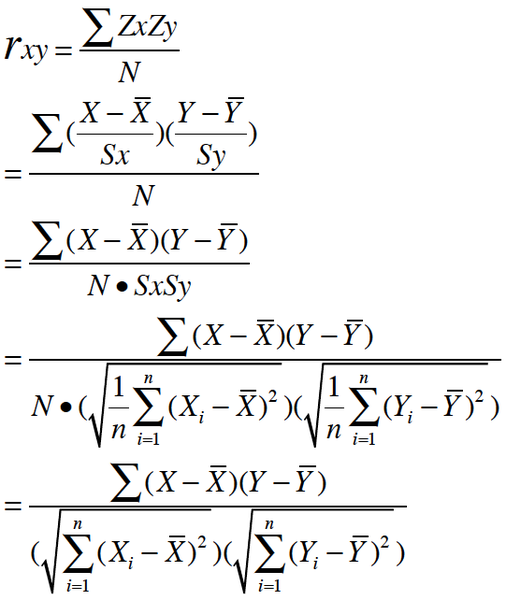# java 皮尔逊相关系数_如何理解皮尔逊相关系数（Pearson Correlation Coefficient）？

Z分数一般代表正态分布中, 数据偏离中心点的距离.等于变量减掉平均数再除以标准差.(就是高考的标准分类似的处理)x的平均数是:3.8

y的平均数是0.138

(1-3.8)*(0.11-0.138)=0.0784

(2-3.8)*(0.12-0.138)=0.0324

(3-3.8)*(0.13-0.138)=0.0064

(5-3.8)*(0.15-0.138)=0.0144

(8-3.8)*(0.18-0.138)=0.1764

0.0784+0.0324+0.0064+0.0144+0.1764=0.308

sum((x-mean(x))^2)=30.8

sum((y-mean(y))^2)= 0.00308

(1-3.8)^2=7.84 #平方

(2-3.8)^2=3.24 #平方

(3-3.8)^2=0.64 #平方

(5-3.8)^2=1.44 #平方

(8-3.8)^2=17.64 #平方

7.84+3.24+0.64+1.44+17.64=30.8

30.8^0.5=5.549775

0.00308^0.5=0.05549775

0.308/(5.549775*0.05549775)=1假设有100人, 一组数据是年龄,平均年龄是35岁,标准差是5岁;另一组数据是发帖数量,平均帖子数量是45份post,标准差是8份帖子.

> y

> cor.test(x,y,method="pearson") #计算这两组数的相关,并进行T检验

data: x and y

t = -0.0269, df = 98, p-value = 0.9786

alternative hypothesis: true correlation is not equal to 0

95 percent confidence interval:

-0.1990316 0.1938019

sample estimates:

cor

-0.002719791

0.6-0.8 强相关

0.4-0.6 中等程度相关

0.2-0.4 弱相关

0.0-0.2 极弱相关或无相关

ps : 这个网站开发者不要再次发明轮子,本来用markdown语法写作很爽,结果又不得不花时间来改动.请考虑尽快支持Markdown语法.

12-1674503-132万+
06-171322
09-111072
12-201537
08-11961
05-135767
12-161412
12-23717
02-1659
06-193812
08-254142
07-214283
10-02706
05-295万+
06-267760